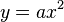# Parameter facts for kids

Kids Encyclopedia Facts

In some non-technical contexts or in jargon, parameter may simply be a synonym for criterion.

In mathematics, statistics, and the mathematical sciences, parameters (L: auxiliary measure) are quantities that define certain relatively constant characteristics of systems or functions.

Most commonly represented θ in general form, other symbols carry standard, specific meanings. When evaluating the function over a domain or determining the response of the system over a period of time, the independent variables are modulated, while the parameters are held constant. The function or system may then be reevaluated or reprocessed with different parameters, to give a function or system with different behavior.

Loosely speaking, the term parameter is used for an argument which is intermediate in status between a variable and a constant.

## Example

• In a section on frequently misused words in his book The Writer's Art, James J. Kilpatrick quoted a letter from a correspondent, giving examples to illustrate the correct use of the word parameter:
 “ W.M. Woods...a mathematician...writes... "...a variable is one of the many things a parameter is not." ... The dependent variable, the speed of the car, depends on the independent variable, the position of the gas pedal. ”
 “ [Kilpatrick quoting Woods] "Now...the engineers...change the lever arms of the linkage...the speed of the car...will still depend on the pedal position...but in a...different manner. You have changed a parameter" ”
• If asked to imagine the graph of the relationship$y= a x^2$, one typically visualizes a range of values of x, but only one value of a. Of course a different value of a can be used, generating a different graphical appearance. The a can therefore be considered to be a parameter: less variable than the variable x, but less constant than the constant 2.
• Suppose you want to buy a bike, and that you believe that the amount of money you are going to make next month is defined by the wage you receive per hour times the amount of hours you are going to work (y = ax)(Income=wage x hours that you work). You are able to influence the amount of hours you work next month (your boss is very flexible), but you think that you will not be able to change the height of your wage (your boss is a real pain when it comes to discussing wages). Then:
• Your wage is the parameter
• The amount of hours you work is the variable.
• This is of course depending on whether you think you can or cannot change your wage.

Suppose that you and a friend are both working for the same boss making the same money working the same hours, that the formula would become:

• (y = 2ax)(Income=2 x wage x hours that you work).
• the constant is 2
• the parameter is your wage
• the variable is the amount of hours you are going to work

### Engineering

In engineering (especially involving data acquisition) the term parameter sometimes loosely refers to an individual measured item. For example, an airliner flight data recorder may record 88 different items, each termed a parameter. This usage is not consistent, as sometimes the term channel refers to an individual measured item, with parameter referring to the setup information about that channel.

"Speaking generally, properties are those physical quantities which directly describe the physical attributes of the system; parameters are those combinations of the properties which suffice to determine the response of the system. Properties can have all sorts of dimensions, depending upon the system being considered; parameters are dimensionless, or have the dimension of time or its reciprocal." John D. Trimmer, 1950, Response of Physical Systems (New York: Wiley), p. 13.

The term can also be used in engineering contexts, however, as it is typically used in the physical sciences. The data that one computer program gives to another are sometimes called parameters.

## Other pagesParameter Facts for Kids. Kiddle Encyclopedia.